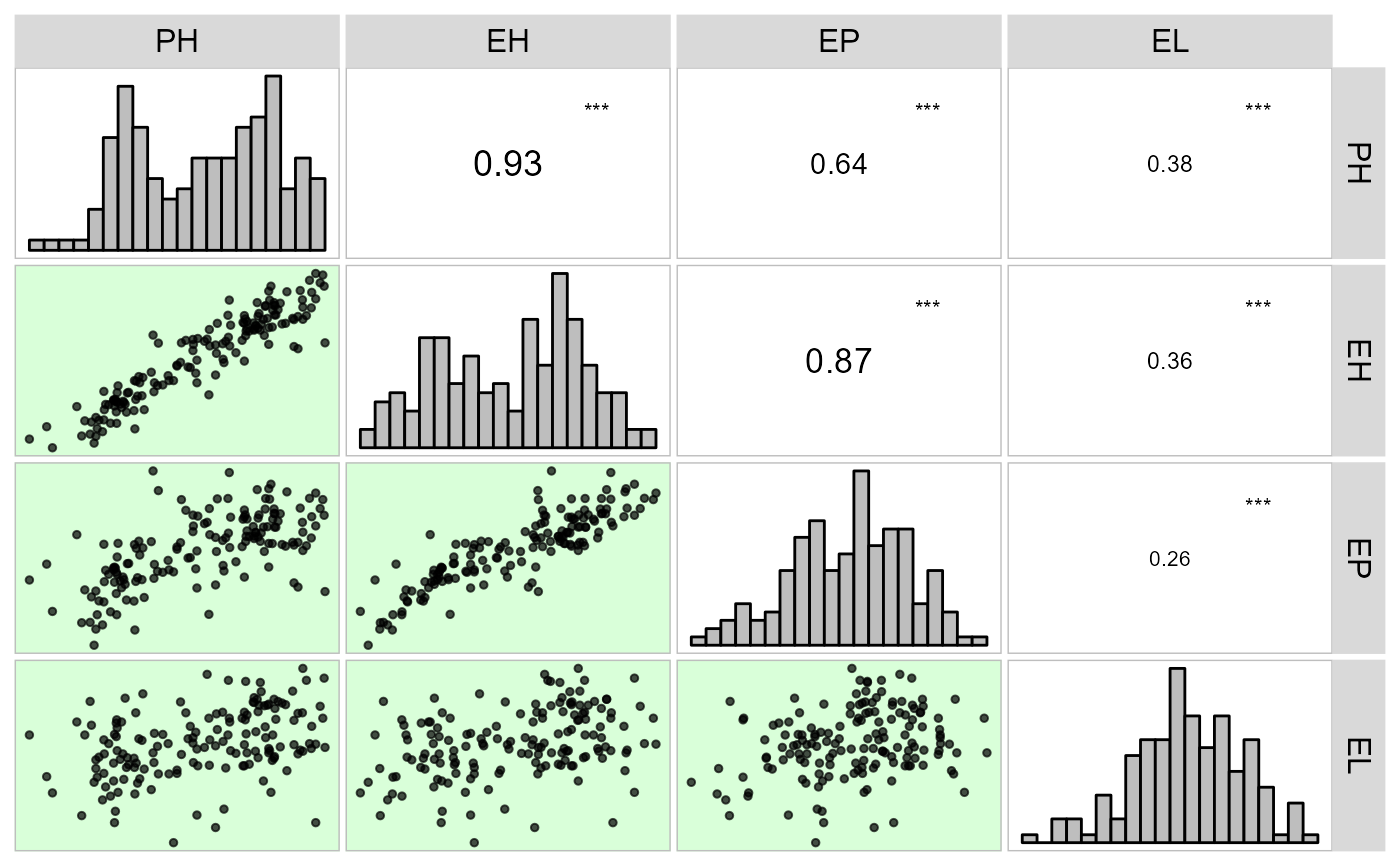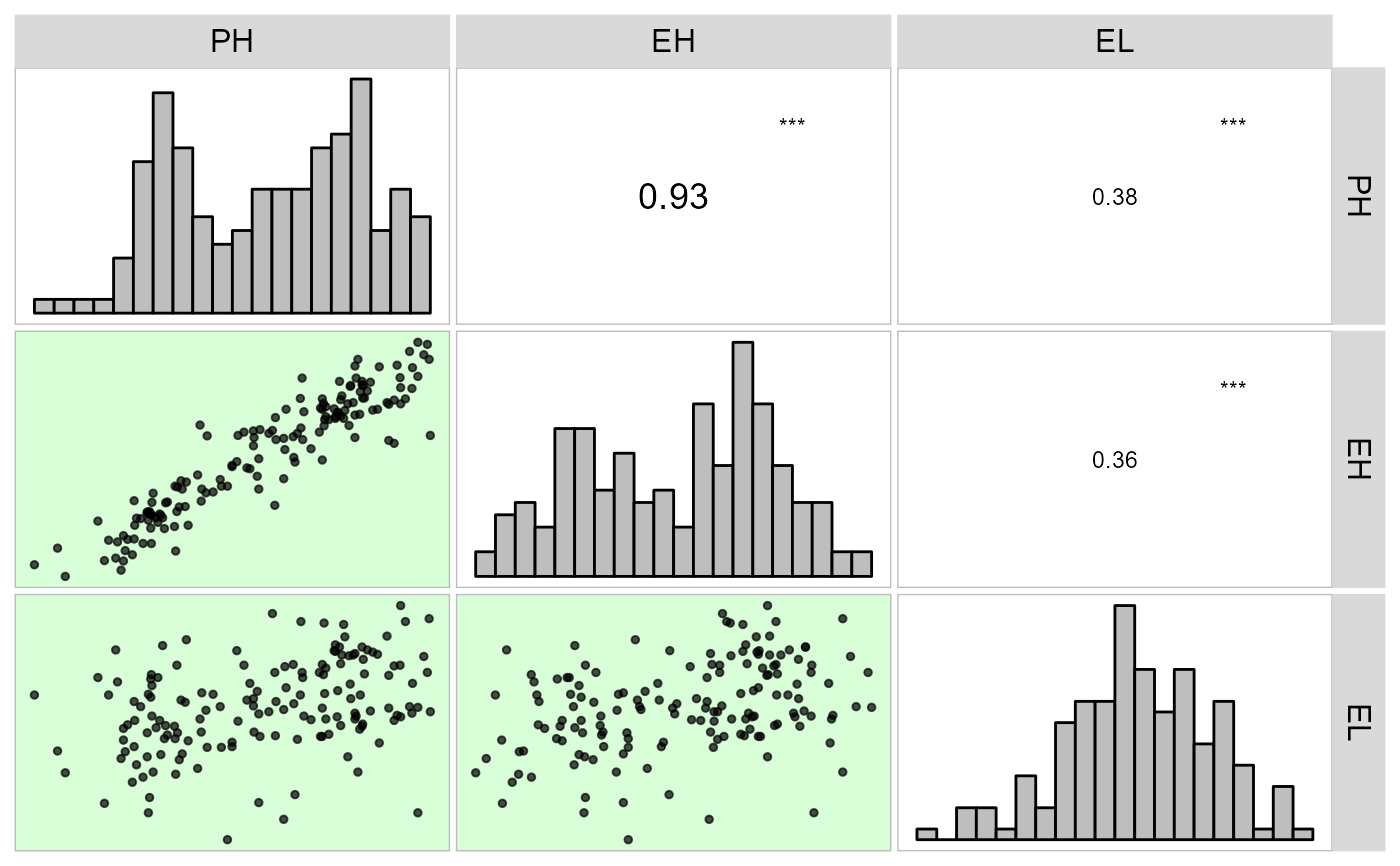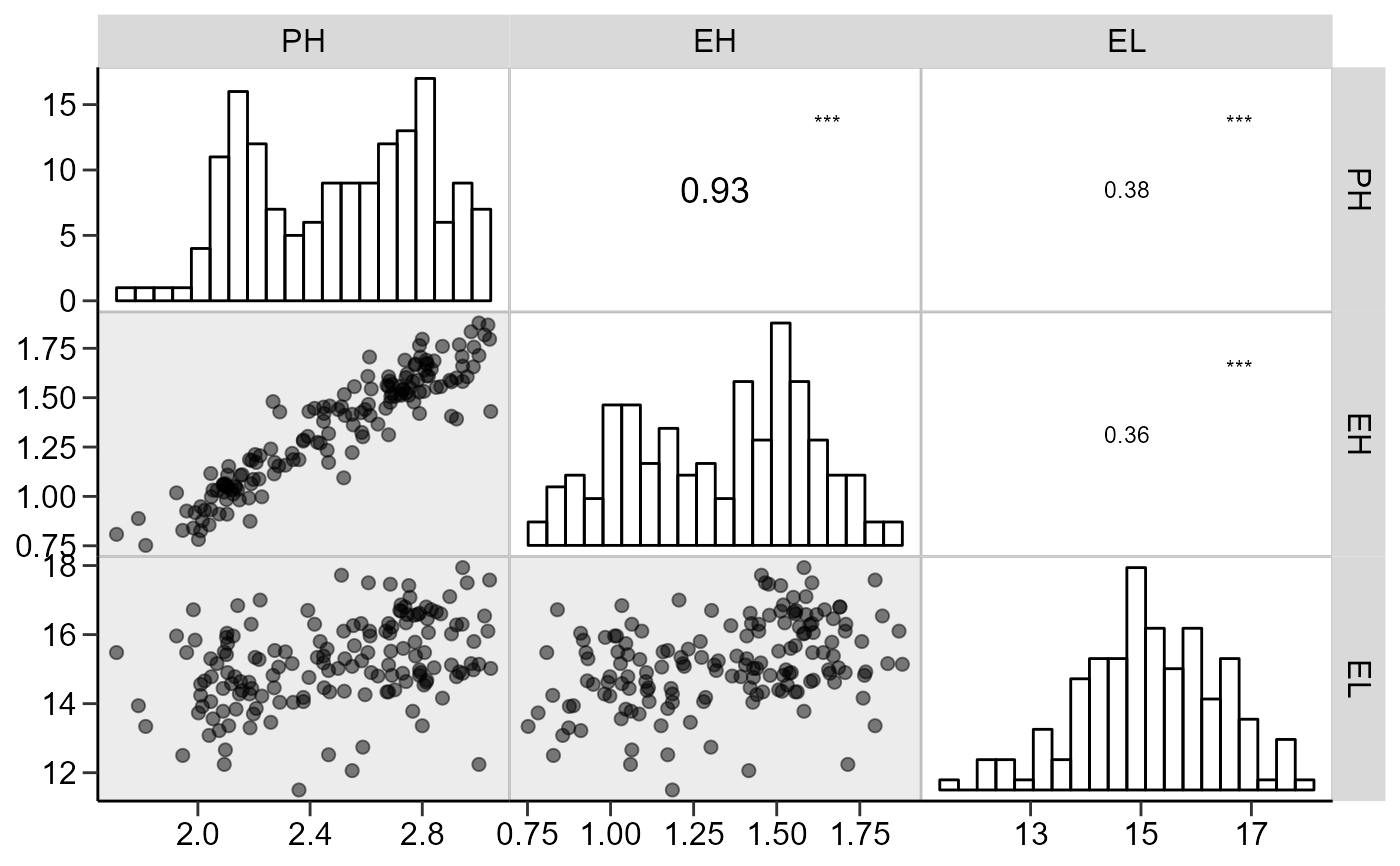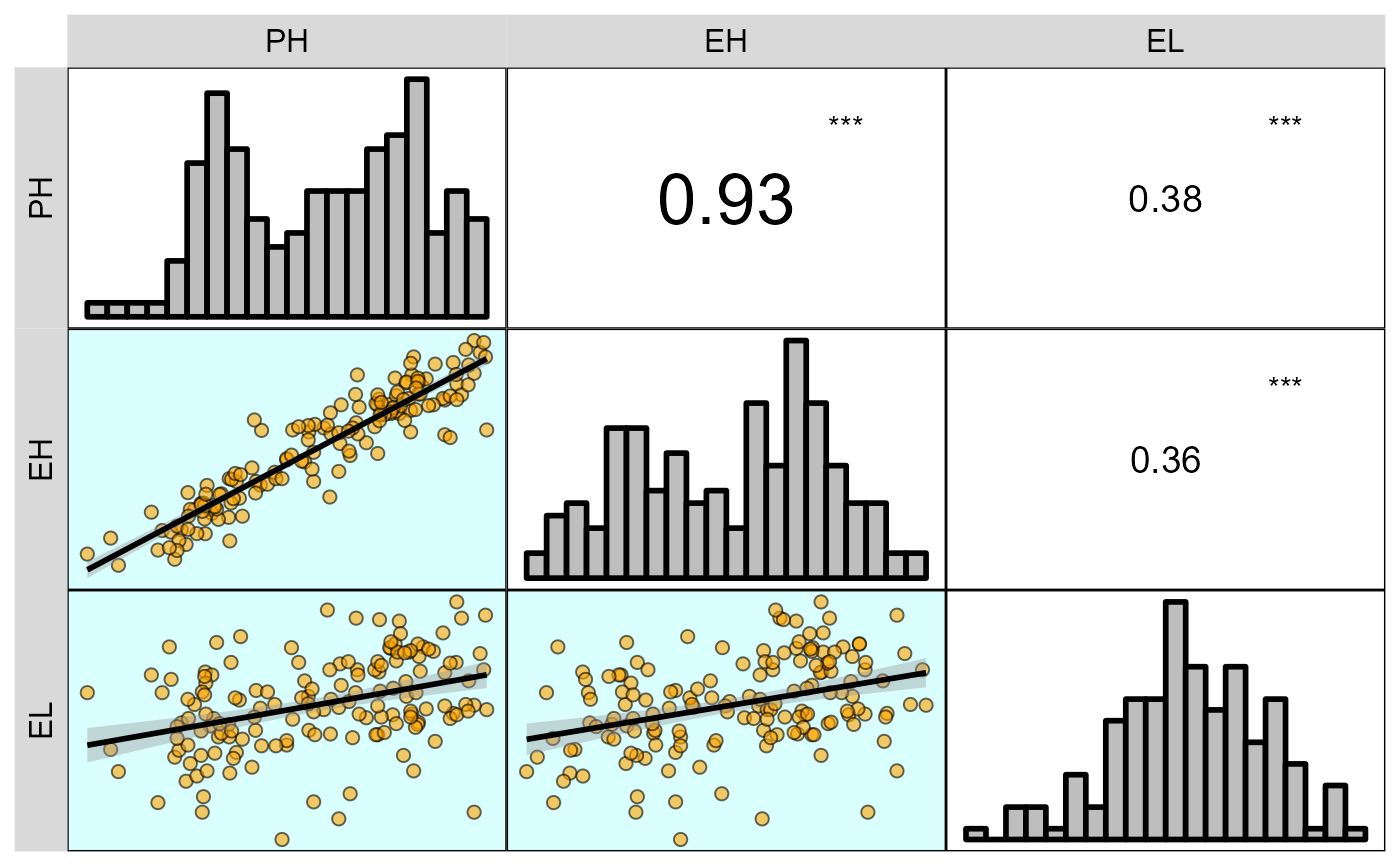Graphical and numerical visualization of a correlation matrix

## Usage

corr_plot(
.data,
...,
col.by = NULL,
upper = "corr",
lower = "scatter",
decimal.mark = ".",
axis.labels = FALSE,
show.labels.in = "show",
size.axis.label = 12,
diag = TRUE,
diag.type = "histogram",
bins = 20,
col.diag = "gray",
alpha.diag = 1,
col.up.panel = "gray",
col.lw.panel = "gray",
col.dia.panel = "gray",
prob = 0.05,
col.sign = "green",
alpha.sign = 0.15,
lab.position = "tr",
progress = NULL,
smooth = FALSE,
col.smooth = "red",
confint = TRUE,
size.point = 1,
shape.point = 19,
alpha.point = 0.7,
fill.point = NULL,
col.point = "black",
size.line = 0.5,
minsize = 2,
maxsize = 3,
pan.spacing = 0.15,
digits = 2,
export = FALSE,
file.type = "pdf",
file.name = NULL,
width = 8,
height = 7,
resolution = 300
)

## Arguments

.data

The data. Should, preferentially, contain numeric variables only. If .data has factor-columns, these columns will be deleted with a warning message.

...

Variables to use in the correlation. If no variable is informed all the numeric variables from .data are used.

col.by

A categorical variable to map the color of the points by. Defaults to NULL.

upper

The visualization method for the upper triangular correlation matrix. Must be one of 'corr' (numeric values), 'scatter' (the scatterplot for each pairwise combination), or NULL to set a blank diagonal.

lower

The visualization method for the lower triangular correlation matrix. Must be one of 'corr' (numeric values), 'scatter' (the scatterplot for each pairwise combination), or NULL to set a blank diagonal.

decimal.mark

The decimal mark. Defaults to ".".

axis.labels

Should the axis labels be shown in the plot? Set to FALSE.

show.labels.in

Where to show the axis labels. Defaults to "show" bottom and left. Use "diag" to show the labels on the diagonal. In this case, the diagonal layer (boxplot, density or histogram) will be overwritten.

size.axis.label

The size of the text for axis labels if axis.labels = TRUE. Defaults to 12.

diag

Should the diagonal be shown?

diag.type

The type of plot to show in the diagonal if diag TRUE. It must be one of the 'histogram' (to show an histogram), 'density' to show the Kernel density, or 'boxplot' (to show a boxplot).

bins

The number of bins, Defaults to 20.

col.diag

If diag = TRUE then diagcol is the color for the distribution. Set to gray.

alpha.diag

Alpha-transparency scale (0-1) to make the diagonal plot transparent. 0 = fully transparent; 1 = full color. Set to 0.15

col.up.panel, col.lw.panel, col.dia.panel

The color for the upper, lower, and diagonal panels, respectively. Set to 'gray'.

prob

The probability of error. Significant correlations will be highlighted with '', '', and '' (0.05, 0.01, and 0.001, respectively). Scatterplots with significant correlations may be color-highlighted.

col.sign

The color that will highlight the significant correlations. Set to 'green'.

alpha.sign

Alpha-transparency scale (0-1) to make the plot area transparent. 0 = fully transparent; 1 = full color. Set to 0.15

lab.position

The position that the labels will appear. Set to 'tr', i.e., the legends will appear in the top and right of the plot. Other allowed options are 'tl' (top and left), 'br' (bottom and right), 'bl' (bottom and left).

progress

NULL (default) for a progress bar in interactive sessions with more than 15 plots, TRUE for a progress bar, FALSE for no progress bar.

smooth

Should a linear smooth line be shown in the scatterplots? Set to FALSE.

col.smooth

The color for the smooth line.

confint

Should a confidence band be shown with the smooth line? Set to TRUE.

size.point

The size of the points in the plot. Set to 0.5.

shape.point

The shape of the point, set to 1.

alpha.point

Alpha-transparency scale (0-1) to make the points transparent. 0 = fully transparent; 1 = full color. Set to 0.7

fill.point

The color to fill the points. Valid argument if points are between 21 and 25.

col.point

The color for the edge of the point, set to black.

size.line

The size of the line (smooth and diagonal).

minsize

The size of the letter that will represent the smallest correlation coefficient.

maxsize

The size of the letter that will represent the largest correlation coefficient.

pan.spacing

The space between the panels. Set to 0.15.

digits

The number of digits to show in the plot.

export

Logical argument. If TRUE, then the plot is exported to the current directory.

file.type

The format of the file if export = TRUE. Set to 'pdf'. Other possible values are *.tiff using file.type = 'tiff'.

file.name

The name of the plot when exported. Set to NULL, i.e., automatically.

width

The width of the plot, set to 8.

height

The height of the plot, set to 7.

resolution

The resolution of the plot if file.type = 'tiff' is used. Set to 300 (300 dpi).

## Value

An object of class gg, ggmatrix.

## Author

Tiago Olivoto tiagoolivoto@gmail.com

## Examples

# \donttest{
library(metan)
dataset <- data_ge2 %>% select_cols(1:7)

# Default plot setting
corr_plot(dataset)# Chosing variables to be correlated
corr_plot(dataset, PH, EH, EL)# Axis labels, similar to the function pairs()
# Gray scale
corr_plot(dataset, PH, EH, EL,
shape.point = 19,
size.point = 2,
alpha.point = 0.5,
alpha.diag = 0,
pan.spacing = 0,
col.sign = 'gray',
alpha.sign = 0.3,
axis.labels = TRUE)corr_plot(dataset, PH, EH, EL,
prob = 0.01,
shape.point = 21,
col.point = 'black',
fill.point = 'orange',
size.point = 2,
alpha.point = 0.6,
maxsize = 4,
minsize = 2,
smooth = TRUE,
size.line = 1,
col.smooth = 'black',
col.sign = 'cyan',
col.up.panel = 'black',
col.lw.panel = 'black',
col.dia.panel = 'black',
pan.spacing = 0,
lab.position = 'tl')
#> geom_smooth() using formula 'y ~ x'
#> geom_smooth() using formula 'y ~ x'
#> geom_smooth() using formula 'y ~ x'# }